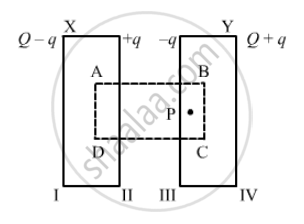Department of Pre-University Education, KarnatakaPUC Karnataka Science Class 12
Share

# The Plates of a Parallel-plate Capacitor Are Given Equal Positive Charges. What Will Be the Potential Difference Between the Plates? What Will Be the Charges - Physics

ConceptCombination of Capacitors

#### Question

The plates of a parallel-plate  capacitor are given equal positive charges. What will be the potential difference between the plates? What will be the charges on the facing surfaces and on the outer surfaces?

#### Solution

It is given that the plates of the capacitor have the same charges. In other words, they are at the same potential, so the potential difference between them is zero.Let us consider that the charge on face II is so that the induced charge on face III is -q and the distribution is according to the figure.

Now, if we consider Gaussian surface ABCD, whose faces lie inside the two plates, and calculate the field at point P due to all four surfaces, it will be

E_1 = (Q-q)/(2∈_0A)

E_2 = (q)/(2∈_0A)

E_3 = (q)/(-2∈_0A)

E_4 = (Q+q)/(2∈_0A)     (It is -Ve because point P is on the left side of face IV. )

Now, as point P lies inside the conductor, the total field must be zero.

therefore E1 + E2 + E3 + E4 = 0

Or

Q-q+q-q+Q+q = 0

∴ q = 0

Hence, on faces II and III, the charge is equal to zero; and on faces I and IV, the charge is Q.
Thus, it seems that the whole charge given is moved to the outer surfaces, with zero charge on the facing surfaces.

Is there an error in this question or solution?

#### APPEARS IN

HC Verma Solution for Concepts of Physics - Vol. 2 (2018 to Current)
Chapter 9: Capacitors
Short Answers | Q: 4 | Page no. 163

#### Video TutorialsVIEW ALL 

Solution The Plates of a Parallel-plate Capacitor Are Given Equal Positive Charges. What Will Be the Potential Difference Between the Plates? What Will Be the Charges Concept: Combination of Capacitors.
S